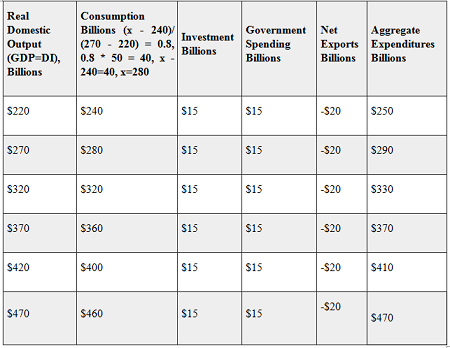# (10 pts.) Complete the table below given the following information: MPC = 0.8 Planned...

## Question:

Complete the table below given the following information:

MPC = 0.8

Planned investment by business is $10 billion when interest rates are 4%, increases by$5 billion for each 1 % decrease in interest rates and the current interest rate is 3%.

Government spending is $15 billion regardless of the level of real domestic output. Exports are$10 billion and imports are $30 billion regardless of the level of real domestic output. Real Domestic Output (GDP=DI), Billions Consumption Billions (x - 240)/ (270 - 220) = 0.8, 0.8 * 50 = 40, x - 240=40, x=280 Investment Billions Government Spending Billions Net Exports Billions Aggregate Expenditures Billions$220 $240$15 -$20$270 $280$15 -$20$320 $320$15 -$20$370 $360$15 -$20$420 $400$15 -$20$470 $460$15 -$20 ## Aggregate Expenditure: Aggregate expenditure is defined as the total value of the goods produced in the economy. It is the aggregate of consumption, investment, government spending and net exports of the economy in a given time period. ## Answer and Explanation: Planned investment is given by$10 billion when interest rates are 4%. With every decrease in 1% of interest rates, investment raises by $5 billion. Therefore, investment at a current interest rate 3% is equal to$15 billion.

Aggregate expenditure is calculated by adding consumption, investment, government spending and net exports.#### Learn more about this topic:Aggregate Supply and Aggregate Demand (AS-AD) Model

from Economics 102: Macroeconomics

Chapter 7 / Lesson 3
58K